## Example Questions

← Previous 1 3 4

### Example Question #1 : Evaluating Expressions

If x = y – 3, then (y – x)=

27

–9

3

–27

9

27

Explanation:

Solve for equation for y – x = 3. Then, plug in 3 into (y – x)= 27.

### Example Question #2 : Evaluating Expressions

When graphed in the (x,y) coordinate plane, at what point do the lines -2x + 4y = 5 and y = -2 intersect?

(13/2,2)

(2,-2)

(-13/2,-2)

(13/2,-2)

(-13/2,-2)

Explanation:

Plugging in y=-2 in the second equation, gives x=-13/2. This is the point where the graphs intersect.

### Example Question #3 : Evaluating Expressions

The length in cm of a plastic container is 5cm less than triple its width.  Which of the following equations is an accurate description of the length, l, as a function of the width, w?

l = 3w + 5

l = 5/3w + 3/5

l = 1/3w – 5

l = 1/3w + 5

l = 3w – 5

l = 3w – 5

Explanation:

This problem requires the development of an equation.  We are told that the length is 5cm less than 3 times its width.  So we should set up an equation that describes this situation. The equation l = 3w – 5 demonstrates how the length is 5 cm less than 3 times the width of the container.

### Example Question #4 : Evaluating Expressions

The expression x(9 + x)(x – 2) = 4 is a polynomial of which degree?

4

1

0

2

3

3

Explanation:

The highest power this polynomial can achieve is 3.

### Example Question #5 : Evaluating Expressions

Given that x = 2 and y = 3, how much less is the value of  3x2 – 2y than the value of  3y– 2x?

17

1

47

29

6

17

Explanation:

First, we solve each expression by plugging in the given values for x and y:

3(22) – 2(3) = 12 – 6 = 6

3(32) – 2(2) = 27 – 4 = 23

Then we find the difference between the first and second expressions’ values:

23 – 6 = 17

### Example Question #6 : Evaluating Expressions

Evaluate 4x+ 6x – 17, when x = 3.

13

36

37

17

30

37

Explanation:

Plug in 3 for x, giving you 36 + 18 – 17, which equals 37.

### Example Question #7 : Evaluating Expressions

John has a motorcycle. He drives it to the store, which is 30 miles away. It takes him 30 minutes to drive there and 60 minutes to drive back, due to traffic. What was his average speed roundtrip in miles per hour?

40 mph

45 mph

50 mph

30 mph

60 mph

40 mph

Explanation:

The whole trip is 60 miles, and it takes 90 minutes, which is 1.5 hours.

Miles per hour is 60/1.5 = 40 mph

### Example Question #8 : Evaluating Expressions

If (xy/2) – 3= –9, what is the value of w in terms of x and y?

3xy – 6

3xy + 6

(1/3)xy + 6

(1/2)xy – 3

w = 3 + (xy/6)

w = 3 + (xy/6)

Explanation:

–3w = –9 – (xy/2)

w = 3 + (xy/6)

### Example Question #9 : Evaluating Expressions

Evaluate 5x+ 16x + 7 when x = 7

365

361

362

364

363

364

Explanation:

Plug in 7 for x and you get 5(49) + 16(7) + 7 = 364

### Example Question #10 : Evaluating Expressions

Let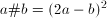for all integersand. Which of the following is the value of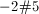?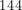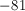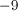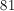Explanation:

In order to solve the expression, replacewith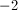andwithin the definition given: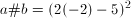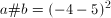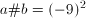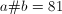← Previous 1 3 4